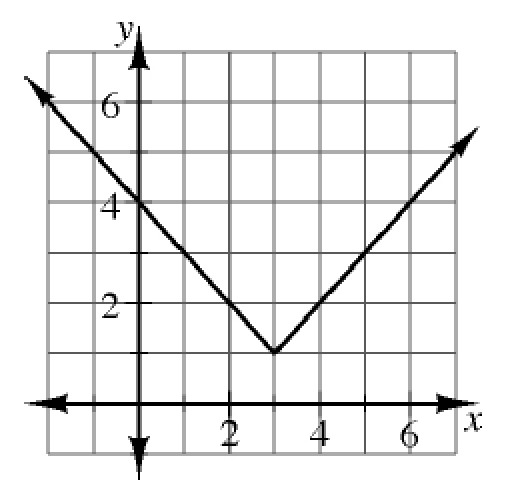### Home > CCA2 > Chapter 3 > Lesson 3.2.5 > Problem3-114

3-114.

Examine the graph of $f(x)=|x-3|+1$ at right. Use the graph to find the values listed below.

1. $f(3)$

What $y$-value corresponds with $x=3$?

$1$

1. $f(0)$

$4$

1. $f(4)$

Refer to parts (a) and (b).

1. $f(-1)$

Refer to parts (a) and (b).